• 生成式 考虑联合分布$P(Y, R, O)$
• 判别式 考虑条件分布$P(Y, R|O)$

• 贝叶斯网，使用有向无环图(常用于变量间存在显式因果关系的情况）
• 马尔科夫网，使用无向图（常用于变量之间存在相关性但没有明显因果性）

## 隐马尔可夫模型

• 观测变量的取值仅依赖于同一时刻的状态变量。
• 当前时刻的状态变量仅依赖于前一时刻的状态变量。即所谓的马尔可夫性质（在DP里叫无后效性）。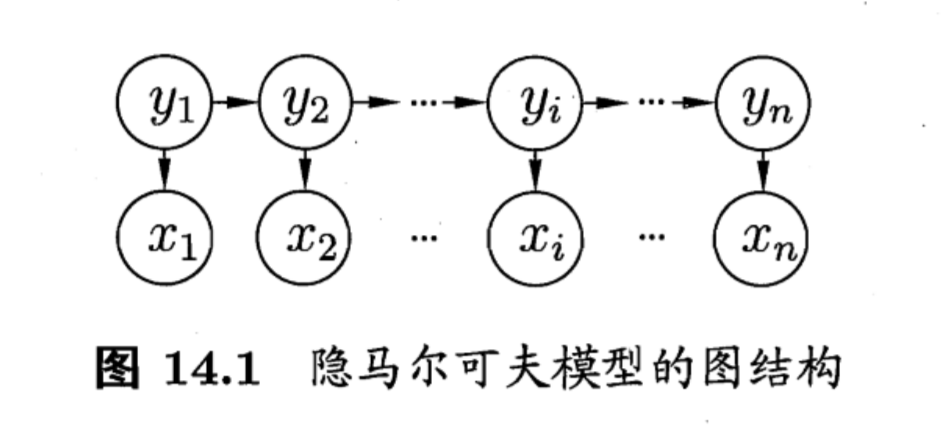• 状态转移概率， 模型在各个状态之间转移的概率，记作$A$
• 输出观测概率，即由状态导出观测的概率分布，记作$B$

• 初始状态概率，模型初始时个状态的概率，记作$\pi$

1. $t=1$时，按照初始状态概率$\pi$选择初始状态$y_1$
2. 由当前状态和输出观测概率$B$选择观测变量$x$
3. 由当前状态和状态转移矩阵$A$确定下一状态
4. 若$t\lt n$，则$t+=1$，并转到第2步，否则停止

• 给定模型参数，如何计算其产生的观测序列的概率的概率$P(X|\lambda)$。
• 给定模型和观测序列，如何找到与该观测序列最匹配的状态序列，即由观测序列推断状态序列。
• 给定观测序列，如何调整模型参数，使得$P(x|\lambda)$最大

## 马尔科夫随机场

• MRF其中有一组势函数(potential function)，又称因子(factor)，它是定义在变量子集上的非负实函数，用于定义概率分布函数。
• 对MRF中节点的自己，若其中任意两个节点之间都有边连接，则称该子集为(clique)，当团中加入任意一个节点都不能构成团，则称其为极大团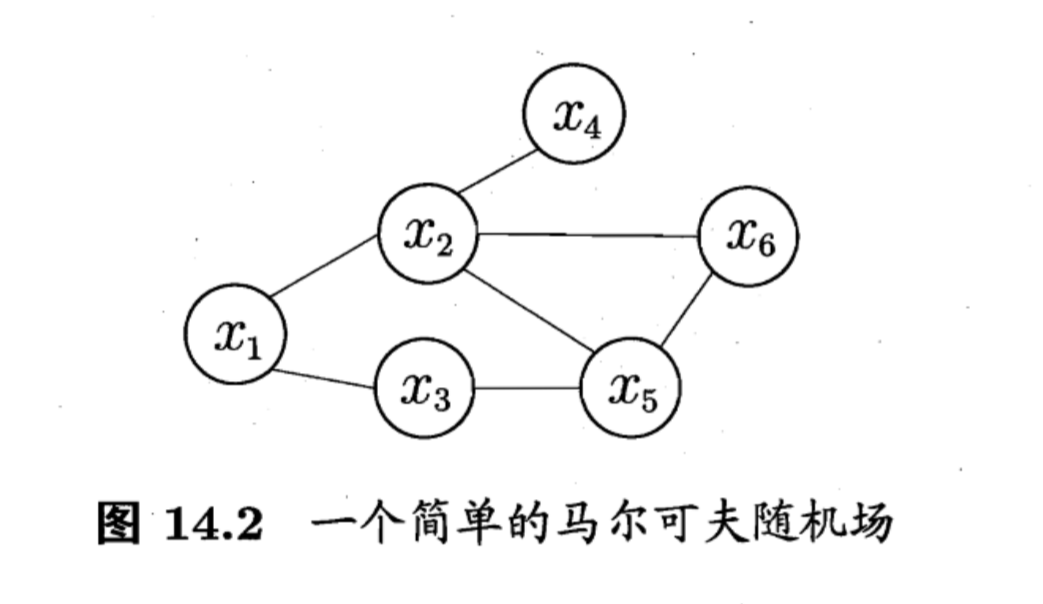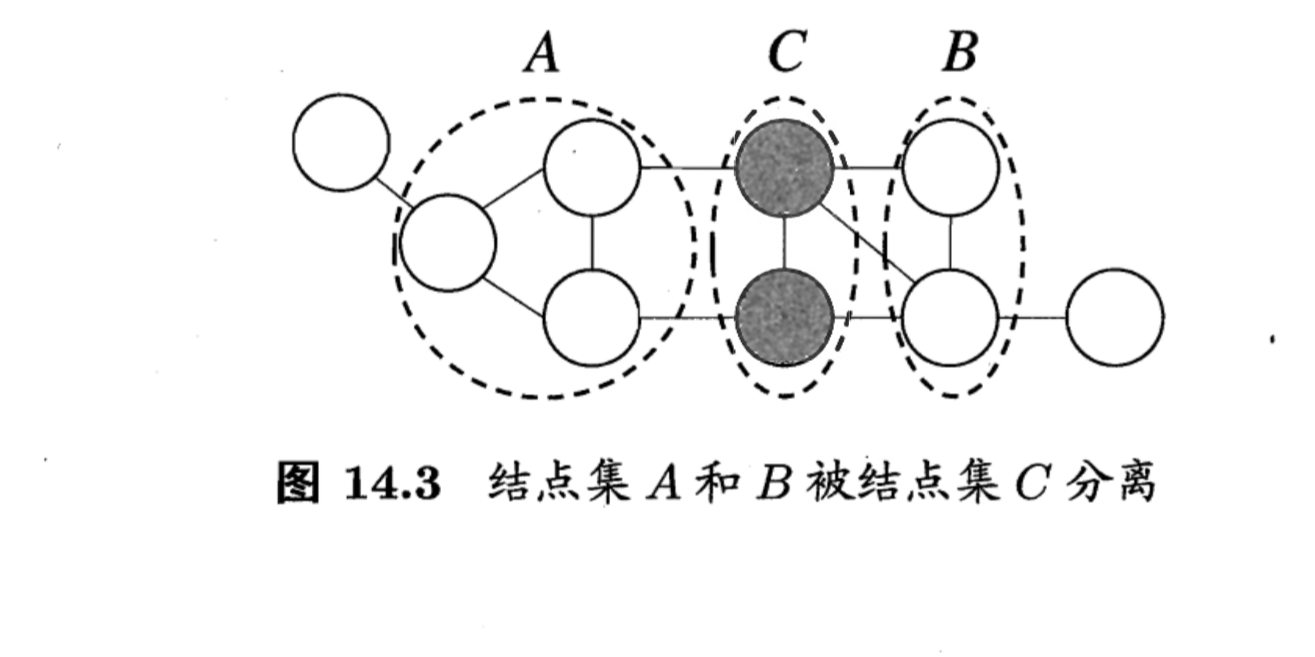• 局部马尔可夫性（local Markov property）：给定某变量的邻接变量，则该变量独立于其他变量
• 成对马尔可夫性（pairwise Markov property）：给定所有其他变量，两个非邻接变量条件独立

MRF中的势函数是用来刻画变量之间的相互关系，为了满足其非负性，势函数被定义为指数函数：

## 条件随机场

CRF的目标是构建条件概率模型$P(y|x)$,标记变量$y$可以是分量之间具有某种相关性的结构型变量（例如词性标注中的序列结构，语法分析中的树形结构）。CRF也利用势函数和团来定义联合概率：

CRF和MRF形式上非常像，区别在于一个处理的是条件概率一个处理的是联合概率。

## 学习和推断

### 信念传播

1. 指定根节点，从所有叶子节点开始向根节点传递消息，直到根节点收到消息。
2. 根节点开始向叶子节点传播消息，直到所有叶子节点收到消息。

## 近似推断

### MCMC采样

#### M-H算法

Metropolis-Hastings算法是MCMC的重要代表，它基于拒绝采样来逼近平稳分布。

1. 基于当前状态的分布进行采样，选出候选样本$x^*$
2. 基于均匀分布采样出阈值$u$
3. 若阈值$u\le A(x^*|x^{t-1})$，则接受该采样结果，否则拒绝该结果。
4. 回到步骤1，直到采样结束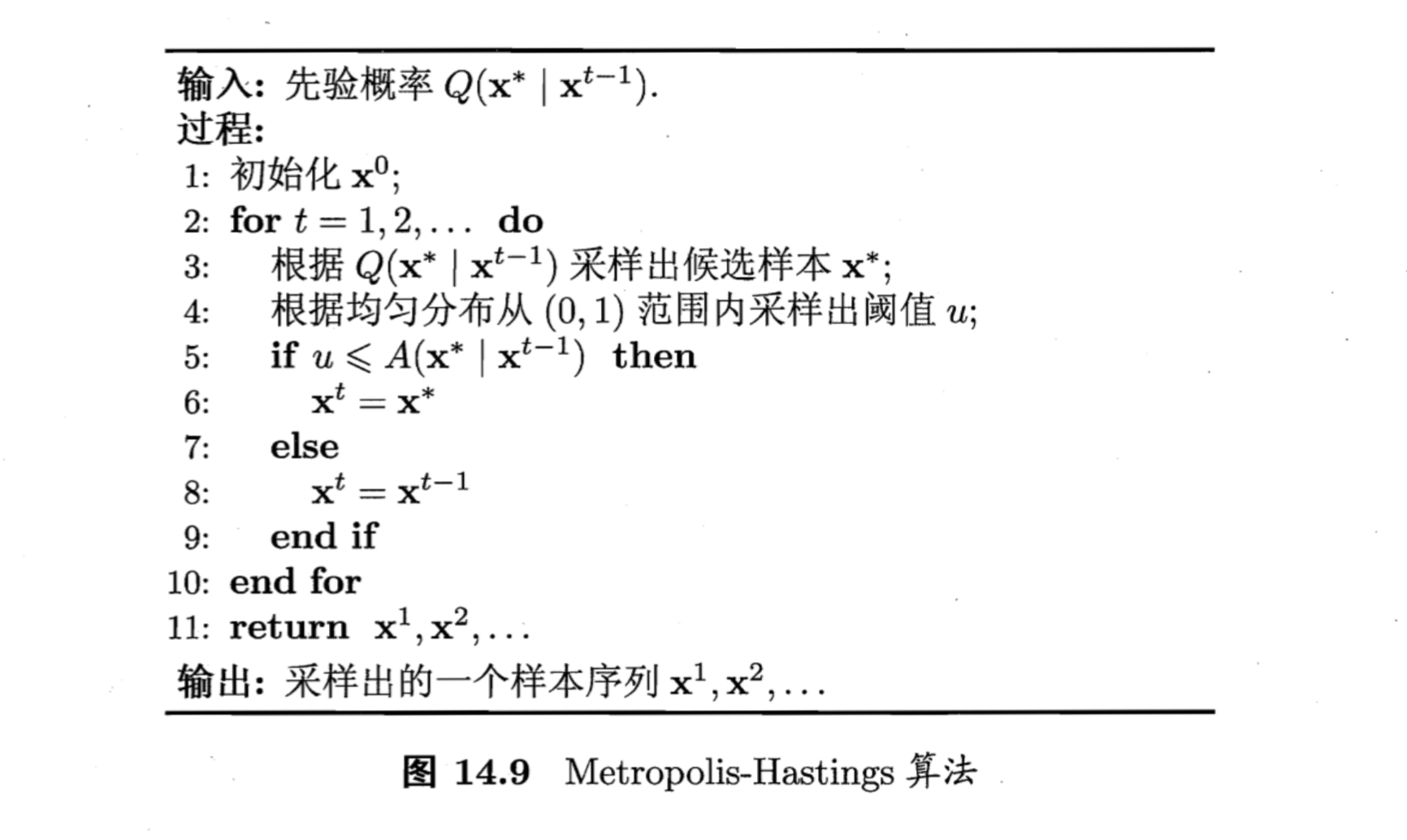### 变分推断

• E-step 固定参数，计算似然函数
• M-step 最大化对数联合似然函数在当前分布下的期望，来更新参数

• 隐变量的分割方式
• 变量子集的分布假设

## 主题模型

1. 根据参数为$\alpha$的迪利克雷分布随机采样一个话题分布$\Theta_t$。
2. 按照以下步骤生成文档中的$N$个词：
• 根据$\Thetat$进行话题指派，得到文档$t$中词$n$的话题$z{t,n}$
• 根据指派的话题所对应的词频分布$\beta_k$随机采样生成词。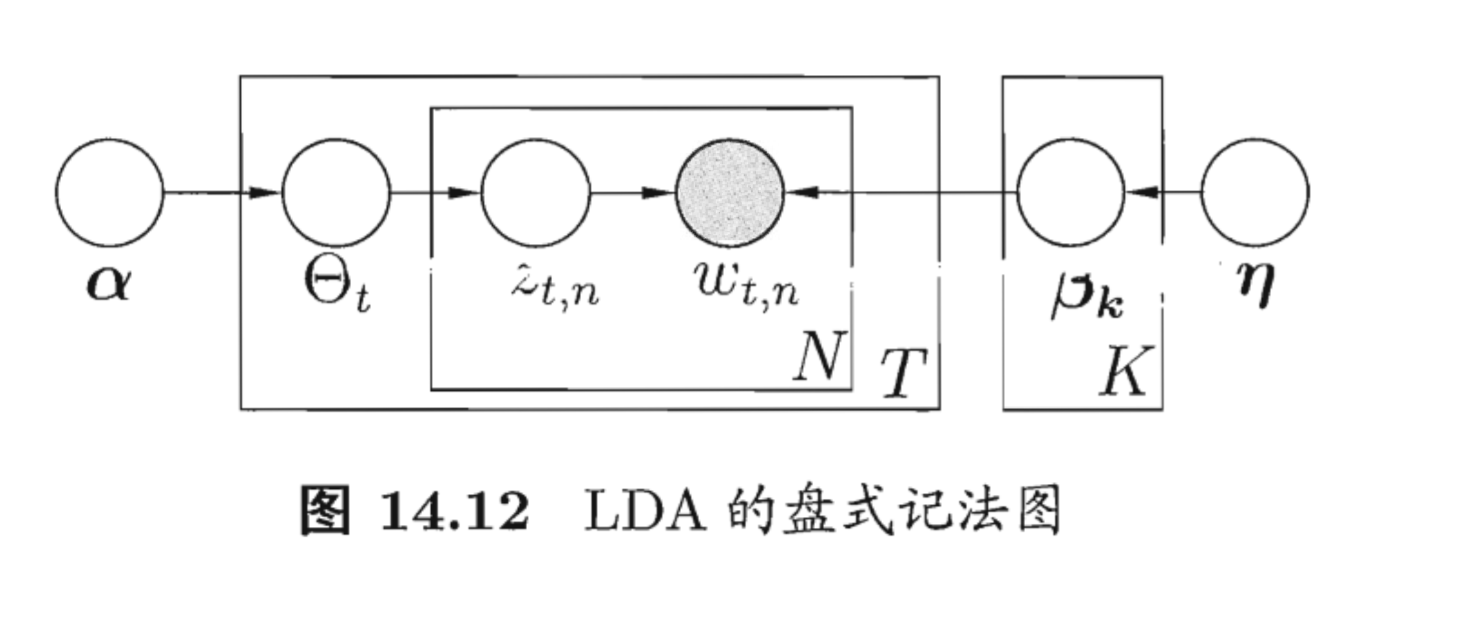## 习题及参考答案

1. 试用盘式记法表示条件随机场和朴素贝叶斯分类器。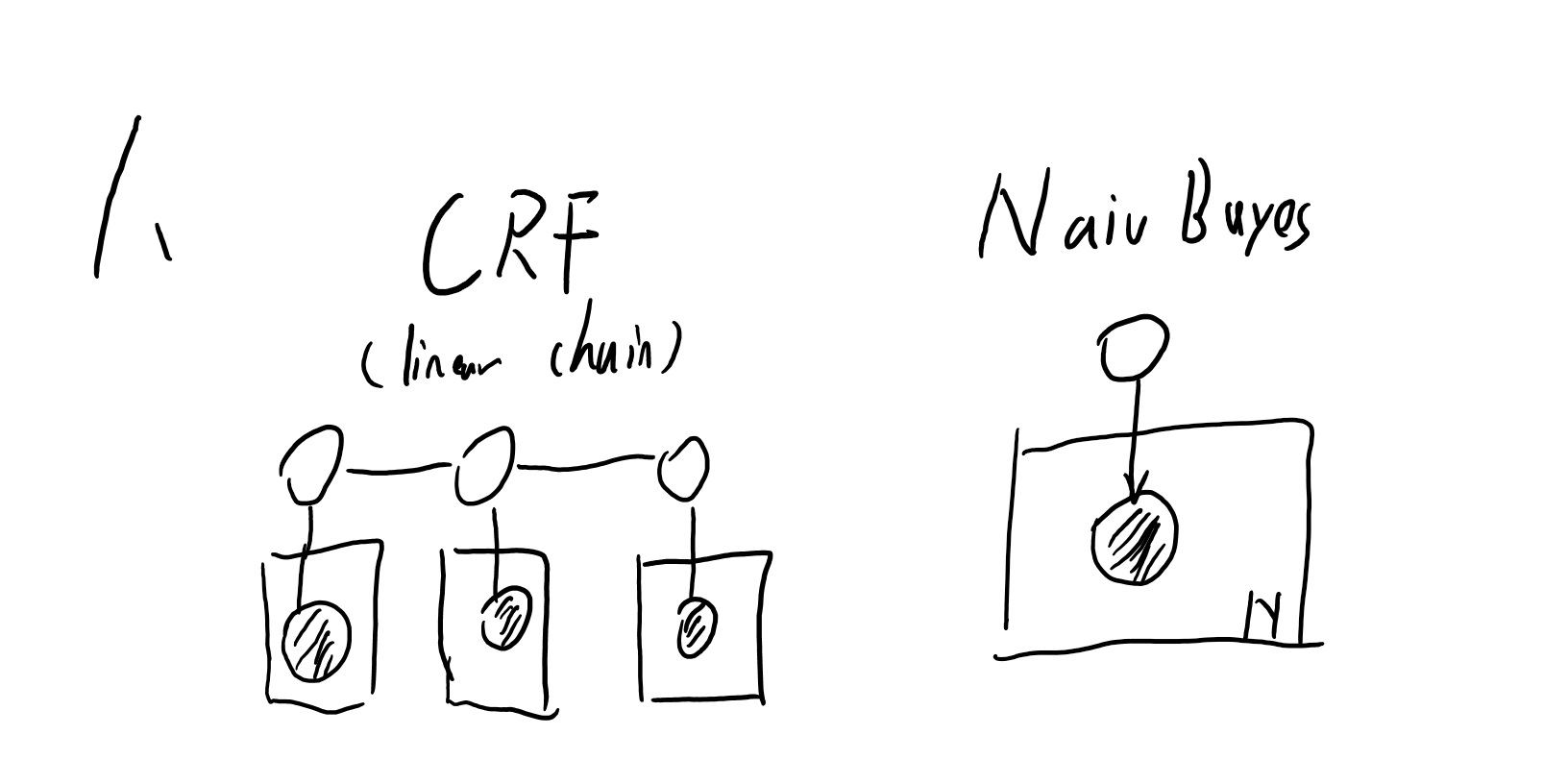2. 试证明图模型中的局部马尔可夫性：给定某变量的邻接变量，则该变量独立于其他变量。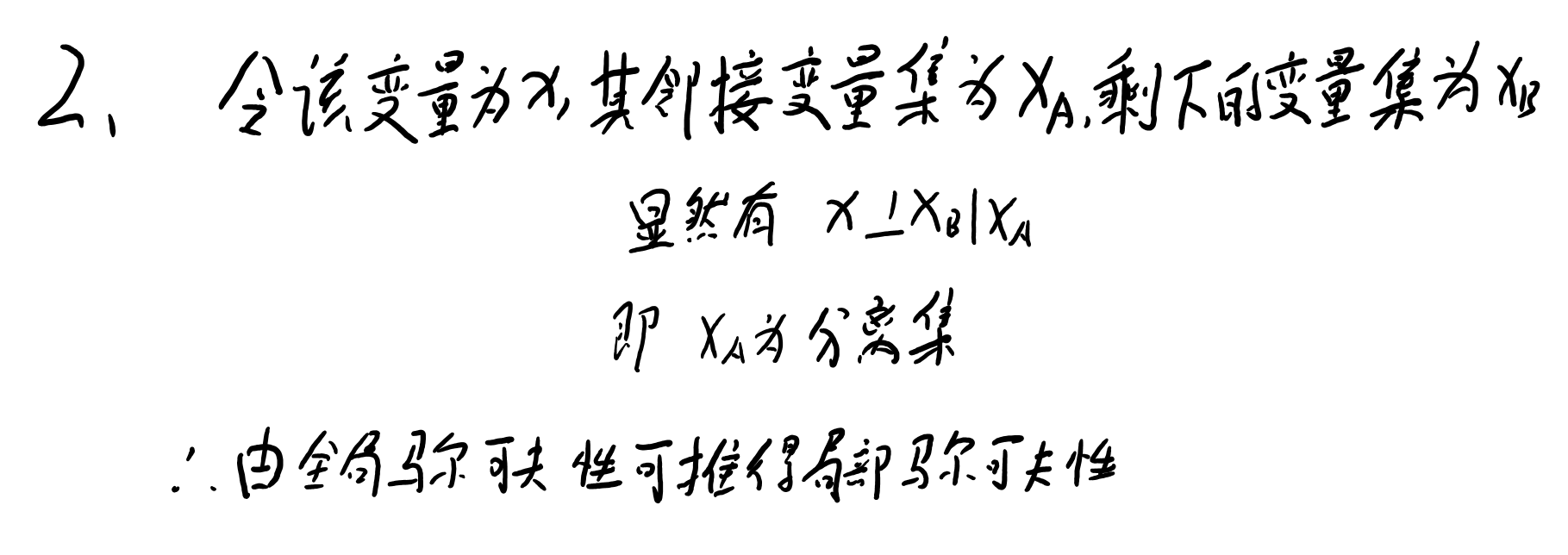3. 试证明图模型中的成对马尔可夫性：给定其他所有变量，则两个非邻接变量条件独立

4. 试述在马尔可夫随机场中为何仅需对极大团定义势函数。

5. 比较条件随机场和对率回归，比较其异同。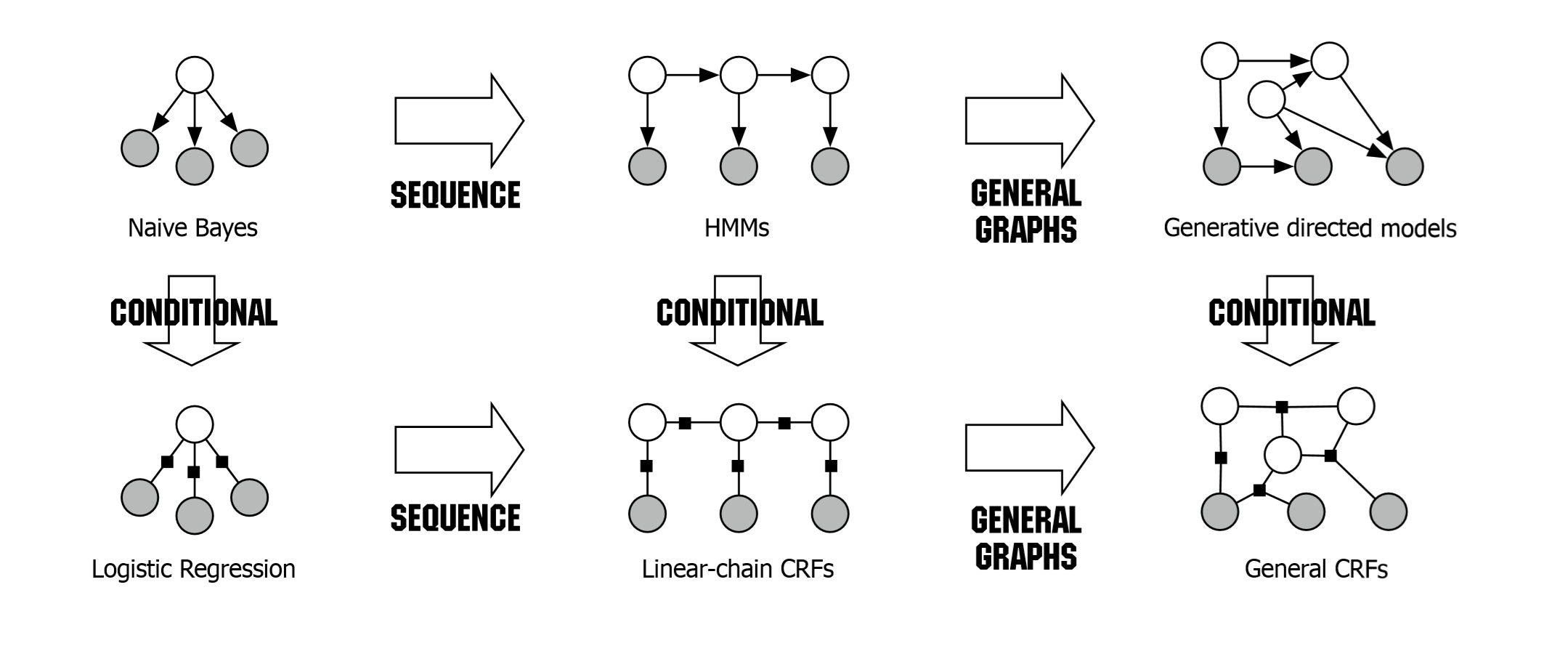6. 试证明变量消去法的计算复杂度随图模型中极大团规模的增长而呈指数增长，但随节点数的增长未必呈指数增长。

7. 吉布斯采样可看作MH算法的特例，但是吉布斯采样中未使用“拒绝采样”策略，试述这样做的好处。

8. 平均场是一种近似推断方法。考虑式(14.32)，试分析平均场方法求解的近似问题与原问题的差异，以及事件中如何选择变量服从的先验分布。

9. 从网上下载或者自己编程实现LDA，试分析金庸作品《天龙八部》中每十回的话题演变情况。

10. 试设计一个无须事先指定话题数目的LDA改进算法。